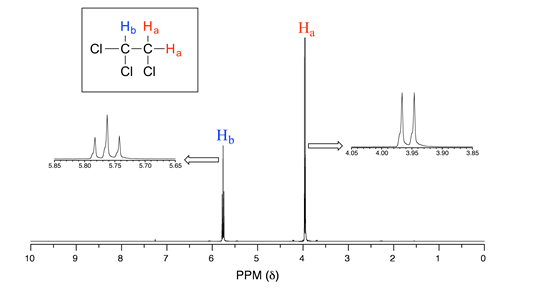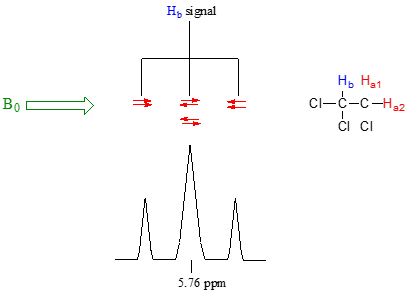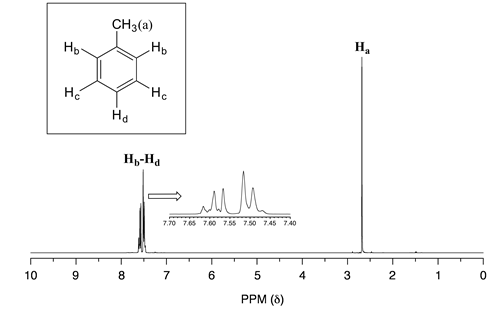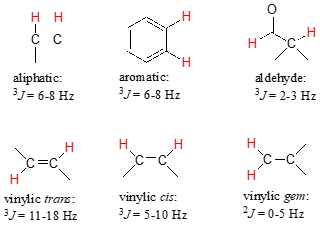# 9.6: Spin-Spin Coupling

$$\newcommand{\vecs}{\overset { \rightharpoonup} {\mathbf{#1}} }$$ $$\newcommand{\vecd}{\overset{-\!-\!\rightharpoonup}{\vphantom{a}\smash {#1}}}$$$$\newcommand{\id}{\mathrm{id}}$$ $$\newcommand{\Span}{\mathrm{span}}$$ $$\newcommand{\kernel}{\mathrm{null}\,}$$ $$\newcommand{\range}{\mathrm{range}\,}$$ $$\newcommand{\RealPart}{\mathrm{Re}}$$ $$\newcommand{\ImaginaryPart}{\mathrm{Im}}$$ $$\newcommand{\Argument}{\mathrm{Arg}}$$ $$\newcommand{\norm}{\| #1 \|}$$ $$\newcommand{\inner}{\langle #1, #2 \rangle}$$ $$\newcommand{\Span}{\mathrm{span}}$$ $$\newcommand{\id}{\mathrm{id}}$$ $$\newcommand{\Span}{\mathrm{span}}$$ $$\newcommand{\kernel}{\mathrm{null}\,}$$ $$\newcommand{\range}{\mathrm{range}\,}$$ $$\newcommand{\RealPart}{\mathrm{Re}}$$ $$\newcommand{\ImaginaryPart}{\mathrm{Im}}$$ $$\newcommand{\Argument}{\mathrm{Arg}}$$ $$\newcommand{\norm}{\| #1 \|}$$ $$\newcommand{\inner}{\langle #1, #2 \rangle}$$ $$\newcommand{\Span}{\mathrm{span}}$$

The $$^1H$$-NMR spectra that we have seen so far (of methyl acetate and 1,4-dimethylbenzene) are somewhat unusual in the sense that in both of these molecules, each set of protons generates a single NMR signal. In fact, the $$^1H$$-NMR spectra of most organic molecules contain proton signals that are 'split' into two or more sub-peaks. Rather than being a complication, however, this splitting behavior is actually very useful because it provides us with more information about our sample molecule.

Consider the spectrum for 1,1,2-trichloroethane. In this and in many spectra to follow, we show enlargements of individual signals so that the signal splitting patterns are recognizable.The signal at 3.96 ppm, corresponding to the two $$H_a$$ protons, is split into two subpeaks of equal height (and area) – this is referred to as a doublet. The $$H_b$$ signal at 5.76 ppm, on the other hand, is split into three sub-peaks, with the middle peak higher than the two outside peaks - if we were to integrate each subpeak, we would see that the area under the middle peak is twice that of each of the outside peaks. This is called a triplet.

The source of signal splitting is a phenomenon called spin-spin coupling, a term that describes the magnetic interactions between neighboring, non-equivalent NMR-active nuclei. (The terms 'splitting' and 'coupling' are often used interchangeably when discussing NMR.) In our 1,1,2 trichloromethane example, the $$H_a$$ and $$H_b$$ protons are spin-coupled to each other. Here's how it works, looking first at the $$H_a$$ signal: in addition to being shielded by nearby valence electrons, each of the $$H_a$$ protons is also influenced by the small magnetic field generated by $$H_b$$ next door (remember, each spinning proton is like a tiny magnet). The magnetic moment of $$H_b$$ will be aligned with $$B_0$$ in slightly more than half of the molecules in the sample, while in the remaining molecules it will be opposed to $$B_0$$. The Beff ‘felt’ by $$H_a$$ is a slightly weaker if $$H_b$$ is aligned against $$B_0$$, or slightly stronger if $$H_b$$ is aligned with $$B_0$$. In other words, in half of the molecules $$H_a$$ is shielded by $$H_b$$ (thus the NMR signal is shifted slightly upfield) and in the other half $$H_a$$ is deshielded by $$H_b$$ (and the NMR signal shifted slightly downfield). What would otherwise be a single $$H_a$$ peak has been split into two sub-peaks (a doublet), one upfield and one downfield of the original signal. These ideas an be illustrated by a splitting diagram, as shown below.Now, let's think about the $$H_b$$ signal. The magnetic environment experienced by $$H_b$$ is influenced by the fields of both neighboring $$H_a$$ protons, which we will call $$H_a1$$ and $$H_a2$$. There are four possibilities here, each of which is equally probable. First, the magnetic fields of both $$H_a1$$ and $$H_a2$$ could be aligned with $$B_0$$, which would deshield $$H_b$$, shifting its NMR signal slightly downfield. Second, both the $$H_a1$$ and $$H_a2$$ magnetic fields could be aligned opposed to $$B_0$$, which would shield $$H_b$$, shifting its resonance signal slightly upfield. Third and fourth, $$H_a1$$ could be with $$B_0$$ and $$H_a2$$ opposed, or $$H_a1$$ opposed to $$B_0$$ and $$H_a2$$ with $$B_0$$. In each of the last two cases, the shielding effect of one $$H_a$$ proton would cancel the deshielding effect of the other, and the chemical shift of $$H_b$$ would be unchanged.So in the end, the signal for $$H_b$$ is a triplet, with the middle peak twice as large as the two outer peaks because there are two ways that $$H_a1$$ and $$H_a2$$ can cancel each other out.

Consider the spectrum for ethyl acetate:We see an unsplit 'singlet' peak at 1.83 ppm that corresponds to the acetyl ($$H_a$$) protons – this is similar to the signal for the acetate protons in methyl acetate that we considered earlier. This signal is unsplit because there are no adjacent protons on the molecule. The signal at 1.06 ppm for the $$H_c$$ protons is split into a triplet by the two $$H_b$$ protons next door. The explanation here is the same as the explanation for the triplet peak we saw previously for 1,1,2-trichloroethane.

The Hb protons give rise to a quartet signal at 3.92 ppm – notice that the two middle peaks are taller then the two outside peaks. This splitting pattern results from the spin-coupling effect of the three adjacent Hc protons, and can be explained by an analysis similar to that which we used to explain the doublet and triplet patterns.

Exercise $$\PageIndex{1}$$

1. Explain, using a splitting diagram, the possible combinations of nuclear spin states for the $$H_c$$ protons in ethyl acetate, and why the $$H_b$$ signal is split into a quartet.
2. The integration ratio of the two 'sub-peaks' in a doublet is 1:1, and in triplets it is 1:2:1. What is the integration ratio of the $$H_b$$ quartet in ethyl acetate? (Hint – use the illustration that you drew in part a to answer this question.)

By now, you probably have recognized the pattern which is usually referred to as the n + 1 rule: if a set of protons has n neighboring, non-equivalent protons, it will be split into n + 1 subpeaks. Thus the two $$H_b$$ protons in ethyl acetate split the $$H_c$$ signal into a triplet, and the three $$H_c$$ protons split the $$H_b$$ signal into a quartet. $$H_a$$, with zero neighboring protons, is a singlet. This is very useful information if we are trying to determine the structure of an unknown molecule: if we see a triplet signal, we know that the corresponding proton or set of protons has two neighbors. When we begin to determine structures of unknown compounds using $$^1H$$-NMR spectral data, it will become more apparent how this kind of information can be used.

Four important points need to be emphasized at this point.

First, signal splitting only occurs between non-equivalent protons – in other words, $$H_a1$$ in 1,1,2-trichloroethane is not split by $$H_a2$$, and vice-versa.Second, splitting occurs primarily between protons that are separated by three or fewer bonds. This is why the $$H_a$$ protons in ethyl acetate form a singlet– the nearest proton neighbors are five bonds away, too far for coupling to occur.With more sensitive instruments we will sometimes see 4-bond and even 5-bond splitting, but in our treatment of NMR, for the sake of simplicity we will always assume that only three-bond splitting is seen.

Third, protons that are bonded to oxygen or nitrogen generally do not split - and are not split by - adjacent protons. $$OH$$ and $$NH$$ protons are acidic enough to rapidly exchange between different molecules, so the neighboring protons never actually 'feels' their influence.

The spectrum of 1-heptanol has a characteristically broad alcohol proton signal at 3.7 ppm (labeled Ha below).Notice in this spectrum that $$H_b$$ is a triplet, coupled to the two $$H_c$$ protons but not coupled to $$H_a$$. The signals corresponding to $$H_c$$ through Hh are complex due to overlapping - when this happens (as it often does!), detailed analysis becomes more challenging.

Below are a few more examples of chemical shift and splitting pattern information for some relatively simple organic molecules.Exercise $$\PageIndex{2}$$

How many proton signals would you expect to see in the $$^1H$$-NMR spectrum of triclosan (a common antimicrobial agent in soap)? For each of the proton signals, predict the splitting pattern, assuming that you can see only 3-bond splitting.Exercise $$\PageIndex{3}$$

How many proton signals would you expect to see in the $$^1H$$-NMR spectrum of the neurotransmitter serotonin? For each of the proton signals, predict the splitting pattern, again assuming only 3-bond splitting.In an ideal world, all NMR spectra would be as easy to interpret as those we have seen so far: every peak would be separated from the others, the peak integration would be obvious, and the multiplicity (singlet, doublet, etc.) would be easy to recognize. The real world, unfortunately, is not always so pretty: peaks with similar chemical shifts overlap, making interpretation much more difficult. We have already seen this is the spectrum of 1-heptanol above. In the spectrum of methylbenzene, we would expect the signal for $$H_a$$ to be a singlet, $$H_b$$ to be a doublet, and $$H_c$$ and $$H_d$$ to be triplets. Looking at relative integration values for the four peaks, we would expect to see a 3:2:2:1 ratio.In practice, however, the three aromatic proton sets $$H_b$$, $$H_c$$ and $$H_d$$ have very similar chemical shifts so their signals overlap substantially, and we cannot recognize doublet or triplet splitting patterns. In this case, we would refer to the aromatic part of the spectrum as a multiplet. We can report the integration ratio of the $$H_a$$ peak compared to the combined aromatic peaks as 3 to 5, or the equivalent 1 to 1.67.

The magnitude of spin-spin coupling can be expressed using the coupling constant, abbreviated with the capital letter J. The coupling constant is simply the difference, expressed in Hz, between two adjacent sub-peaks in a split signal, and is a measure of the extent to which one nucleus 'feels' the magnetic dipole of its neighbor.

For our doublet in the 1,1,2-trichloroethane spectrum, for example, the two subpeaks are separated by 6.1 Hz, and thus we write $$^3J_{a-b} = 6.1 Hz$$.The superscript '3' tells us that this is a three-bond coupling interaction, and the 'a-b' subscript tells us that we are talking about coupling between $$H_a$$ and $$H_b$$. Unlike the chemical shift value, the coupling constant, expressed in Hz, is the same regardless of the applied field strength of the NMR magnet. The strength of the magnetic moment of a neighboring proton, which is the source of the spin-spin coupling phenomenon, does not depend on the applied field strength. For this reason, coupling constants are normally given in Hz, not ppm.

When we look closely at the triplet signal in 1,1,2-trichloroethane, we see that the coupling constant - the 'gap' between subpeaks - is 6.1 Hz, the same as for the doublet. The coupling constant $$^3J_{a-b}$$ quantifies the magnetic interaction between the Ha and Hb hydrogen sets, and this interaction is of the same magnitude in either direction. In other words, spin-spin coupling is reciprocal: $$H_a$$ influences $$H_b$$ to the same extent that $$H_b$$ influences $$H_a$$.

Coupling constants between proton sets on neighboring $$sp^3$$-hybridized carbons is typically in the region of 6-8 Hz. Coupling constants for neighboring vinylic protons can range from 0 Hz (no coupling at all) to 18 Hz, depending on the bonding arrangement.

Typical proton-proton coupling constantsFor vinylic protons in a trans configuration, we see coupling constants in the range of $$^3J = 11-18 Hz$$, while cis protons couple in the $$^3J = 5-10 Hz$$ range. The 2-bond coupling between protons on the same alkene carbon (referred to as geminal protons) is very fine, generally 5 Hz or lower.

Fine coupling (2-3 Hz) is often seen between an aldehyde proton and a three-bond neighbor.

Exercise $$\PageIndex{4}$$

Give the expected splitting patterns and approximate coupling constants for the labeled protons in the compound below.

## Presenting NMR data in table format

Information from NMR can be recorded conveniently in a condensed form without having to reproduce the actual spectrum. For example, the information from the $$^1H$$-NMR specta of ethyl acetate and methylbenzene (see earlier figures) can be presented in tabular format, listing the chemical shift, the peak splitting pattern, and the relative area under peaks (usually, the smallest peak is set to 1). Coupling constant information is not shown in the example tables below.

Table $$\PageIndex{1}$$: $$^1H$$-NMR spectrum of ethyl acetate
ppm splitting integration
3.92 q 1
1.83 s 1.5
1.06 t 1.5
Table $$\PageIndex{2}$$: $$^1H-NMR$$ spectrum of methylbenzene.
ppm splitting integration
7.45 - 7.63 m 1.67
2.64 s 1

(abbreviations: s = singlet, d = doublet, t = triplet, q = quartet, m = multiplet)

Exercise $$\PageIndex{5}$$

Match the $$^1H$$-NMR spectrum below to its corresponding compound, and assign all of the signals.a) cyclopentanone b) 3-pentanone c) butanal

d) 2-pentanone e) 4-heptanone f) 1-butene

Exercise $$\PageIndex{6}$$

1. Which of the compounds in the previous exercise is expected to have an $$^1H$$-NMR spectrum consisting of two triplets and a sextet?
2. Which would have a spectrum consisting of two triplets?
3. Which would have a spectrum that includes a signal above 8 ppm?

Exercise $$\PageIndex{7}$$

Explain how you could distinguish among the $$^1H$$-NMR spectra of the three isomers below.Video tutorials: proton NMR spectroscopy

Video of an actual NMR experiment

## Contributors

9.6: Spin-Spin Coupling is shared under a CC BY-NC-SA license and was authored, remixed, and/or curated by Tim Soderberg.